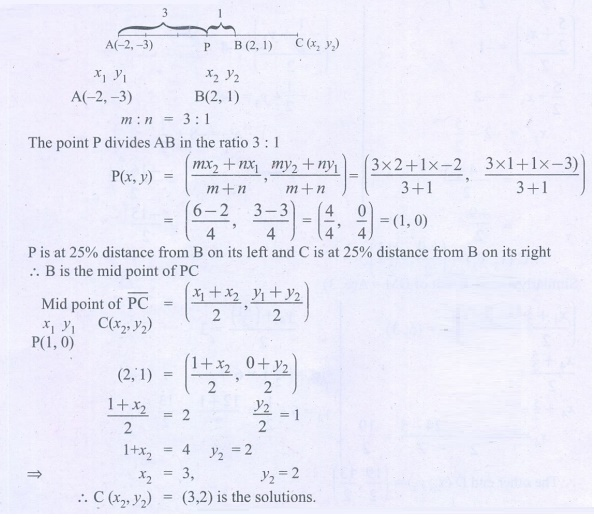Home | | Maths 9th std | Exercise 5.4: Points of Trisection of a Line Segment, Section Formula

# Exercise 5.4: Points of Trisection of a Line Segment, Section Formula

Maths : Coordinate Geometry: Book Back, Exercise, Example Numerical Question with Answers, Solution: Exercise 5.4: Points of Trisection of a Line Segment, Section Formula

Coordinate Geometry

Points of Trisection of a Line Segment, Section Formula

Exercise 5.4

1. Find the coordinates of the point which divides the line segment joining the points A(4, 3) and B(9, 7) in the ratio 3:2.2. In what ratio does the point P(2, 5) divide the line segment joining A(3, 5) and B(4, 9) .3. Find the coordinates of a point P on the line segment joining A(1, 2) and B(6, 7) in such a way that AP = 2/5 AB.4. Find the coordinates of the points of trisection of the line segment joining the points A(5, 6) and B(4, 3) .5. The line segment joining A(6,3) and B(−1, −4) is doubled in length by adding half of AB to each end. Find the coordinates of the new end points.6. Using section formula, show that the points A(7, −5), B(9, −3) and C(13,1) are collinear.7. A line segment AB is increased along its length by 25% by producing it to C on the side of B. If A and B have the coordinates (2, 3) and (2,1) respectively, then find the coordinates of C.Tags : Numerical Problems with Answers, Solution | Coordinate Geometry | Maths , 9th Maths : UNIT 5 : Coordinate Geometry
Study Material, Lecturing Notes, Assignment, Reference, Wiki description explanation, brief detail
9th Maths : UNIT 5 : Coordinate Geometry : Exercise 5.4: Points of Trisection of a Line Segment, Section Formula | Numerical Problems with Answers, Solution | Coordinate Geometry | Maths